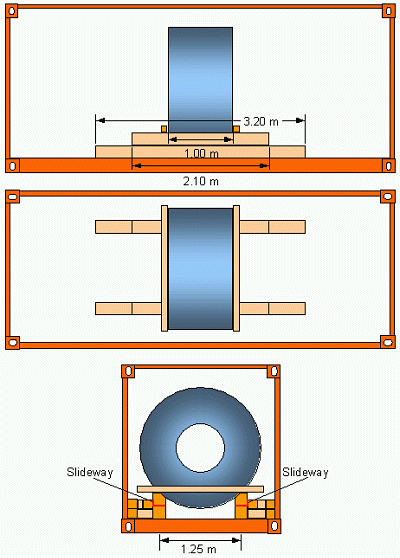6.4   Weight class HIn this example one coil of 17 t shall be loaded into a container of P = 28.230 t. Thus, the coil has a mass of 60 % of the payload. The diameter is d = 1.86 m and the breadth is b = 1.00 m. The coil is tendered without skid and shall be placed "Eye fore and aft". For bedding the coil, square timbers of 20 x 20 cm cross-section are allocated, on which the coils is pushed into the container by means of soft soap. The section modulus of these timbers is 1217 cm³. The diagram in Figure 16 shows, with a load of 8.5 t per beam, a permissible extension of about 1.1 m and hence a length of the beam of t = 2.1 m. This particular length requires a spread of the longitudinal beams of 1.6 m, according to the diagram in Figure 13. This spread cannot be realised with a coil diameter of 1.86 m. The maximum possible spread of 70 % of that diameter is 1.30 m. This however, requires a beam length t = 3.10 m. Eventually, a spread of s = 1.25 m with a length of beams t = 3.20 m is selected according to the diagram in Figure 13. The permissible extension of 1.1 m is maintained by laying shorter beams of 2.1 m length in between. The arrangement is shown in Figure 31.Figure 31: Longitudinal stowage of a "heavy" coilContact  |  Site Map  |  Glossary  |  Legal Notice